• 基于Matlab直线检测直线检测基于Matlab直线检测直线检测
• 基于Matlab直线检测基于Matlab直线检测基于Matlab直线检测
• 写在前面的话：文章内容来源于但不限于网络、书籍、个人心得体会等，意在总结和方便各位同行快速参考，共同学习进步，记录自己的问题。错误在所难免，有请各位批评斧正。如有侵权，烦请第一时间通知，我会立即删除...
写在前面的话：文章内容来源于但不限于网络、书籍、个人心得体会等，意在总结和方便各位同行快速参考，共同学习进步，记录自己的问题。错误在所难免，有请各位批评斧正。如有侵权，烦请第一时间通知，我会立即删除相关内容，万分感谢！

Hough变换小程序，拿去学习吧，时间久了，可能有错误。

I= imread('2.png');
BW1=im2bw(I,0.95);
BW2=double(BW1);
BW3=edge(BW2,'sobel');
[H,T,R]=hough(BW3);
imshow(H,[],'XData',T,'YData',R,'InitialMagnification','fit');
xlabel('\theta');ylabel('\rho');
axis on,axis normal,hold on;
P=houghpeaks(H,5,'threshold',ceil(0.3*max(H(:))));
x=T(P(:,2));y=R(P(:,1));
plot(x,y,'s','color','white');
lines=houghlines(BW3,T,R,P,'FillGap',5,'MinLength',7);
figure,imshow(BW3),hold on;
In images.internal.initSize (line 71)
In imshow (line 336)
max_len=0;
for k=1:length(lines);
xy=[lines(k).point1;lines(k).point2];
plot(xy(:,1),xy(:,2),'lineWidth',2,'Color','green');
plot(xy(1,1),xy(1,2),'x''lineWidth',2,'Color','yellow');
plot(xy(2,1),xy(2,2),'x''lineWidth',2,'Color','red');
len=norm(lines(k).point1-lines(k).point2);
if(len>max_len)
max_len=len;
xy_long=xy;
end
end
plot(xy_long(:,1),xy_long(:,2),'LineWidth',2,'Color','cyan');


展开全文• 使用matlab进行RANSAC直线检测。在一堆随机点中隐藏一条直线，通过RANSAC方法检测出来。RANSAC方法不保证每次都能找到最优的直线。尝试的次数越多，成功的概率越大。
• matlab实现的hough变换直线检测，单独的.m文件，方便插入调用。
• LSD直线检测算法首先计算图像中所有点的梯度大小和方向，然后将梯度方向变化小且相邻的点作为一个连通域，接着根据每一个域的矩形度判断是否需要按照规则将其断开以形成多个矩形度较大的域，最后对生成的所有的域做...
一、简介
LSD是一种线段检测算法^{}，该方法能在较短的时间内获得较高精度的直线段检测结果。
LSD直线检测算法首先计算图像中所有点的梯度大小和方向，然后将梯度方向变化小且相邻的点作为一个连通域，接着根据每一个域的矩形度判断是否需要按照规则将其断开以形成多个矩形度较大的域，最后对生成的所有的域做改善和筛选，保留其中满足条件的域，即为最后的直线检测结果。该算法的优点在于检测速度快，而且无需参数调节，利用错误控制的方法，提高直线检测的准确度。
1  算法介绍
LSD直线检测方法首先计算每个像素点的水平线（Level-Line）角度，从而形成了一个水平线场（Level-Line Field），即单位矢量场。这里像素点的水平线角度就是该点梯度方向的垂直角度，如图2-1所示，而水平线场就是一个与图像中的点一一对应的矩阵，矩阵中元素的值即为对应到图像中点的水平线角度，如图2-2中间图所示。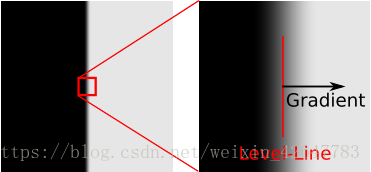获得水平线场后，依据水平线角度用区域生长的方法将其切割成若干个连通域，每个连通域中所有像素点的水平线角度变化不能超过容忍值\tau，这样的连通域称为线支持区域（Line Support Regions），每个线支持区域都是线段检测的候选对象。如图2所示，绿色区域、橙色区域和蓝色区域各是一个线支持区域。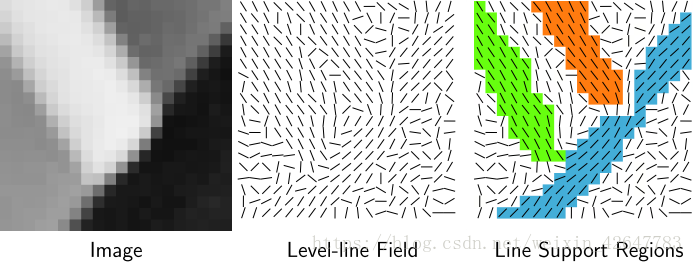获得线支持区域后，把线支持区域的主惯性轴方向作为矩形方向，构造一个包含区域中所有点的矩形，如图2-3所示构造方式在3.6节中详细说明。然后将矩形中所有水平线方向角度与矩形方向角度偏差小于\tau的点叫做对齐点（Aligned Point）如图2-4所示，设一个矩形内总的点数为n，其中对齐点点数为k，这将用于之后验证矩形是否能作为线段检测结果。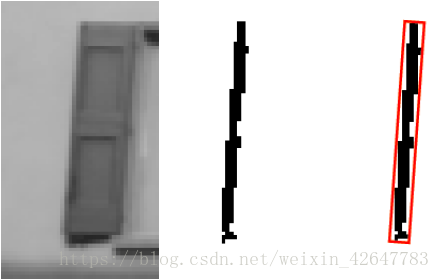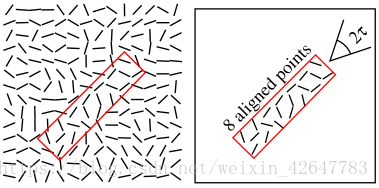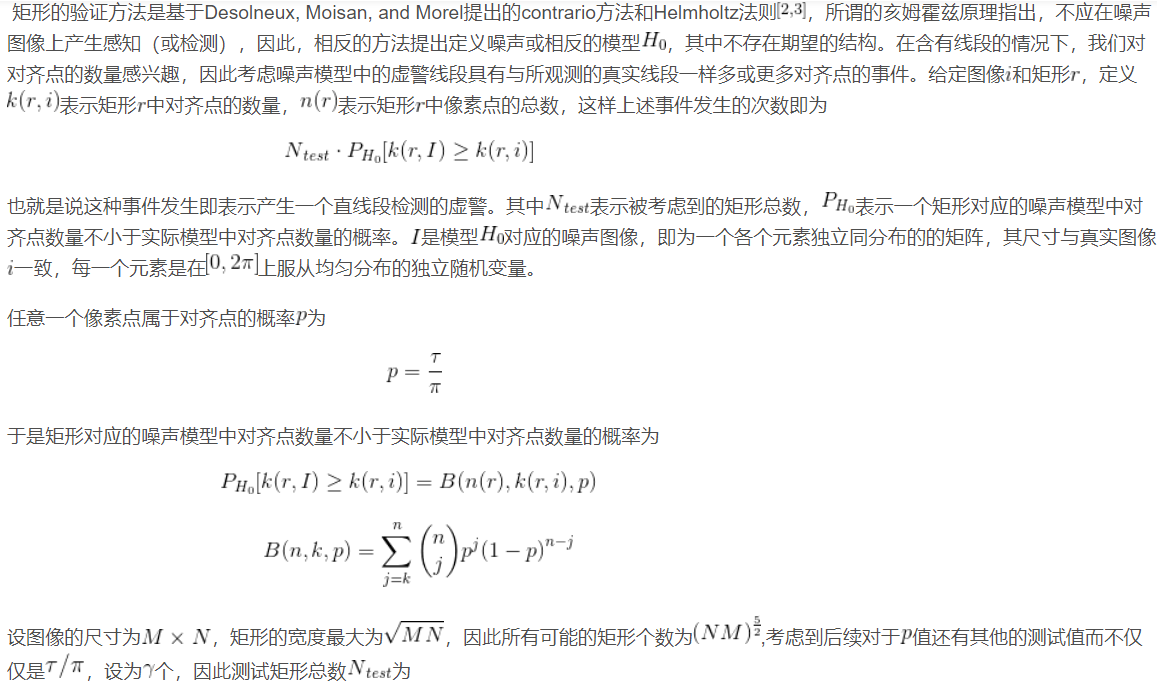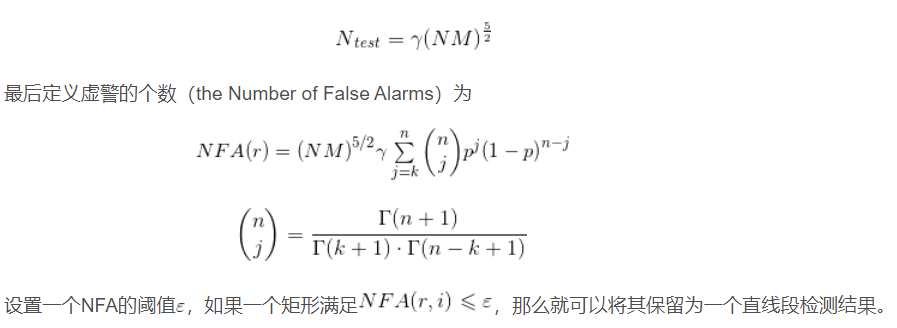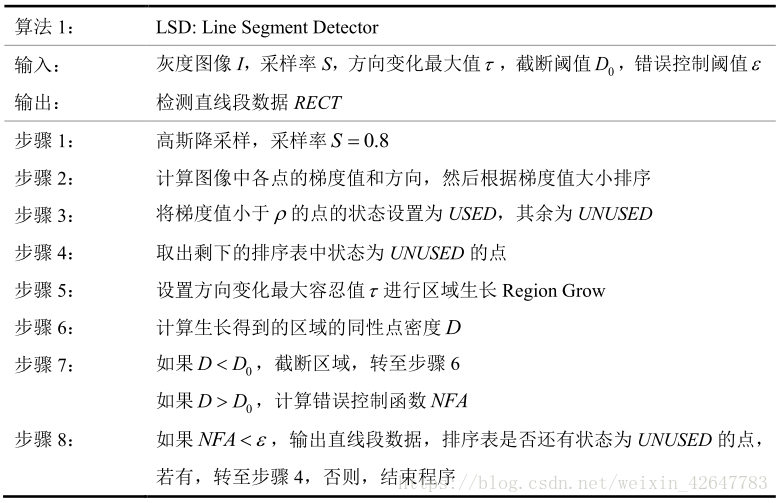二、源代码
% lsd store and draw
img_path = './undistortedImage/1.png';

a = detect(img_path);
lines_list = flsd(a);
lines_list = lines_list(:,1:4);
swap = lines_list(:,1);
lines_list(:,1) = lines_list(:,2);
lines_list(:,2) = swap;
swap = lines_list(:,3);
lines_list(:,3) = lines_list(:,4);
lines_list(:,4) = swap;
% lines_list = and;
fusion_lines = mergeLine(lines_list,5,5,10,180);
% minAngleDis = 5; minDis = 5; minLen = 20; minGap = 180;

img = imread(img_path);
line = fusion_lines;
figure,
hold on
imagesc(img);
colormap bone;
for i = 1: size(line,1)
plot([line(i,1),line(i,3)],[line(i,2),line(i,4)],'red');
end
axis ij
hold off

img = imread(img_path);
line = lines_list;
figure,
hold on
imagesc(img);
colormap bone;
for i = 1: size(line,1)
plot([line(i,1),line(i,3)],[line(i,2),line(i,4)],'red');
end
axis ij
hold off

三、运行结果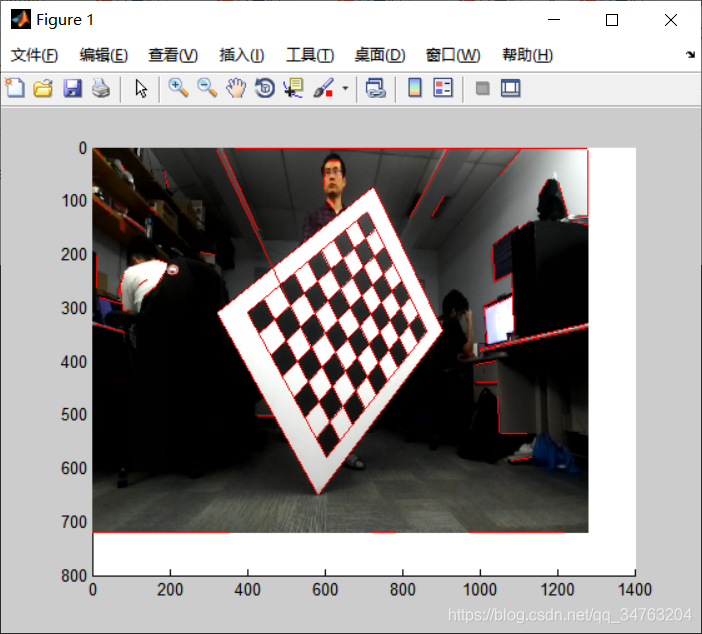四、备注
版本：2014a


展开全文• Matlab实现 霍夫变换直线检测
分享一下我老师大神的人工智能教程！零基础，通俗易懂！http://blog.csdn.net/jiangjunshow也欢迎大家转载本篇文章。分享知识，造福人民，实现我们中华民族伟大复兴！                霍夫变换实现直线检测——MATLAB网上好多不能用，就重新写了一个：% 入口图像为 BW，出口图像为fclc,closeBW=imread('D:\picture\9dafa605d53eea243812bb29.jpg');BW=rgb2gray(BW);thresh=[0.01,0.17];sigma=2;%定义高斯参数f = edge(double(BW),'canny',thresh,sigma);figure(1),imshow(f,[]);title('canny 边缘检测');[H, theta, rho]= hough(f, 0.5);%imshow(theta,rho,H,[],'notruesize'),axis on,axis normal%xlabel('\theta'),ylabel('rho');[r,c]=houghpeaks(H,5);hold onlines=houghlines(f,theta,rho,r,c);figure,imshow(f,[]),title('Hough Transform Detect Result'),hold onfor k=1:length(lines)     xy=[lines(k).point1;lines(k).point2];plot(xy(:,1),xy(:,2),'LineWidth',4,'Color',[.6 .6 .6]);endMatlab2012版本的内核函数有所改变，houghpeaks, houghlines等函数有所改变，感谢cuicd2011的提醒，R2012a版本的直线检测稍有变化：clc,close  BW=imread('D:\picture\9dafa605d53eea243812bb29.jpg');  BW=rgb2gray(BW);  thresh=[0.01,0.17];  sigma=2;%定义高斯参数  f = edge(double(BW),'canny',thresh,sigma);  figure(1),imshow(f,[]);  title('canny 边缘检测');    [H, theta, rho]= hough(f,'RhoResolution', 0.5);  %imshow(theta,rho,H,[],'notruesize'),axis on,axis normal  %xlabel('\theta'),ylabel('rho');    peak=houghpeaks(H,5);  hold on    lines=houghlines(f,theta,rho,peak);  figure,imshow(f,[]),title('Hough Transform Detect Result'),hold on  for k=1:length(lines)      xy=[lines(k).point1;lines(k).point2];      plot(xy(:,1),xy(:,2),'LineWidth',4,'Color',[.6 .6 .6]);  end  实验结果：给我老师的人工智能教程打call！http://blog.csdn.net/jiangjunshow展开全文• 此为一个直线检测MATLAB源程序，经实验可以运行，很有帮助，顶一下！
• 1、Hough检测直线原理 Hough变换目的 原始图像中直线检测问题转化为寻找参数空间中的交点个数统计的极值问题。 基本原理 在r-theta参数空间中具有同样（theta，r）的点在一条直线上。 直角坐标系中无法表达垂直...
本文简单介绍Hough原理，重点整理matlab的Hough工具箱——Hough/houghpeaks/houghlines函数
1、Hough检测直线原理
Hough变换目的
原始图像中直线的检测问题转化为寻找参数空间中的交点个数统计的极值问题。
基本原理
在r-theta参数空间中具有同样（theta，r）的点在一条直线上。
直角坐标系中无法表达垂直直线，极坐标系中，每一条直线都有一条过笛卡尔坐标系坐标原点的垂线，且对于每条直线来说，这条垂线是唯一的，而这条垂线，我们就用（theta, r）来表示，其中theta为该垂线与x轴所成的夹角，r 为该垂线的长度（原点到直线的距离）。所以可以用（theta，r）唯一表示一条直线。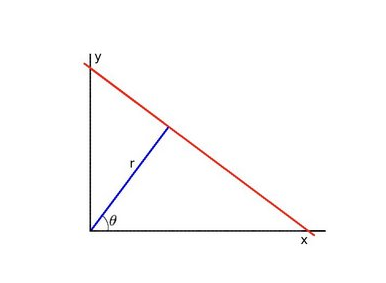也就是说图像空间中的一条线，在r-theta参数空间中为一个点，经过某一点的所有直线为r-theta参数空间中的一条曲线（三角函数曲线），r-theta参数空间中曲线的交点为图像中一条直线，r-theta参数空间中的一条水平直线为图像空间中的一个圆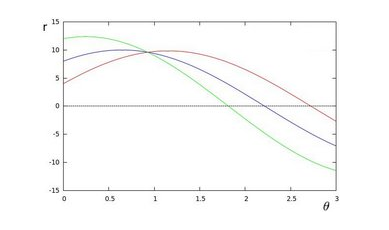具体检测方法
对于图像的每个像素点（x，y），对应到参数空间中，找到曲线的交点，交于此点的曲线个数就是图像空间的直线上的像素点数。
设置一个阈值，交于一点曲线个数超过这个值，图像中才算一条直线。
2、matlab工具箱
首先对图像进行边缘提取，然后对边缘进行Hough变换提取直线。
hough
对二值边缘图像进行Hough变换
[H,theta,rho] = hough(BW,Name,Value,...)

输入：
BW：边缘提取后的二值图的图像矩阵
可设置参数：‘RhoResolution’和’Theta’
RhoResolution：垂线长度的变化步长（对于数字图像，垂线的原点为左上角）
缺省值：1，取值范围：0~norm（size（BW））
Theta：过每一像素点的直线的旋转角度范围和步长
缺省值：-90:1:89
输出：
H：hough变换矩阵，（一行表示一垂线长度，一列表示一旋转角度）矩阵中的值表示过（theta, rho）的曲线的个数，即直线上像素点个数
theta：角度
rho：垂线长度
houghpeaks
提取后变换后的极值点
peaks = houghpeaks(H,numpeaks，Name,Value,...)

输入：
H：hough 变换矩阵
numpeaks：极值点个数
缺省值：1
可设置参数：‘Threshold’和’NHoodSize’
threshold：可以提取的最小极值
缺省值：最大极值的一半0.5*(max(H(：)))
NhoodSize：抑制区域的大小，即该区域内只取一个极值点（该参数的值为奇数数组）
缺省值：size(H)/50，或者>size(H)/50的奇数
输出：
peaks：参数空间中的极值点的坐标
houghlines
lines = houghlines(BW,theta,rho,peaks,Name,Value,...)

输入：
BW:边缘图
theta：与上面的hough算出来的theta一致
rho：与上面的hough算出来的一致
peaks：与上面的houghpeaks算出来的一致
可设置参数：‘FillGap’和’MinLength’
FillGap：具有相同的垂线长度（rho相同）的两根直线之间首尾距离小于FillGap的，可以认为是同一条直线
缺省值：20
MinLength：直线长度<MinLength 的舍去
缺省值：40
输出：
lines：为structure变量，包含直线的起点、终点、角度垂线、长度。
3、完整hough变换检测直线程序
close all; clear ;clc
I  = imread('scratch.tif');
figure;
subplot(1,3,1);
imshow(I,[]);
BW = edge(I,'canny');%Canny方法提取图像边界，返回二值图像(边界1,否则0)
[H,T,R] = hough(BW,'RhoResolution',0.5,'Theta',-10:0.5:10);%计算二值图像的标准霍夫变换，H为霍夫变换矩阵，I,R为计算霍夫变换的角度和半径值
subplot(1,3,2);
imshow(H,[],'XData',T,'YData',R,'InitialMagnification','fit');%hough变换的图像
xlabel('\theta'), ylabel('\rho');
axis on,axis square,hold on;

P  = houghpeaks(H,3,'threshold',0.7*max(H(:)));%提取3个极值点
x = T(P(:,2));
y = R(P(:,1));
plot(x,y,'s','color','white');%标出极值点

lines=houghlines(BW,T,R,P);%提取线段
subplot(1,3,3);
imshow(I), hold on;
for k = 1:length(lines)
xy = [lines(k).point1; lines(k).point2];
plot(xy(:,1),xy(:,2),'LineWidth',2,'Color','green');%画出线段
plot(xy(1,1),xy(1,2),'x','LineWidth',2,'Color','yellow');%起点
plot(xy(2,1),xy(2,2),'x','LineWidth',2,'Color','red');%终点
end



展开全文• 在实现matlab时，其中有一些需要二值化处理的地方，由于图像是植物，这里根据绿色的地方RGB通道中绿色通道的数值会较大的特点进行二值化。
• 网上找到的。想了解Hough霍夫曼直线检测效果的，可以下来学习
• 基于hough变换检测直线，用MATLAB实现 我只是想换积分下个东西。。。hough
• 基于hough变换的直线检测matlab代码，可直接运行，无需配置
• radon变换直线检测matlab代码检测针状晶体图像上的线 该存储库包含一些MATLAB函数和一个演示脚本，我们用于检测图像上的针状晶体以监控其生长。 方法的结果和说明可以在中找到。 如果您使用此代码进行研究，请...
• 数字图像处理 Hough 变换直线检测 ,matlab 实现 实验八 Hough 变换直线检测 一实验目的 理解Hough变换的原理了解其应用掌握利用Hough变换进行直线检测的处 理过程 及编程方法 二实验内容 利用Hough变换检测直线通常...
• 关于Radon变换的原理网上有很多介绍了，这里根据radon变化的结果得到检测出的直线。以一条直线为例： 首先对图片进行处理：srcImage=imread(fileName);grayImage=rgb2gray(srcImage);cannyImage=edge(grayImage,'...
• LSD直线检测算法首先计算图像中所有点的梯度大小和方向，然后将梯度方向变化小且相邻的点作为一个连通域，接着根据每一个域的矩形度判断是否需要按照规则将其断开以形成多个矩形度较大的域，最后对生成的所有的域做...
• 实验要求，生成5条直线并添加噪声，使用霍夫变换拟合直线 霍夫变换原理请参考此博客：... 实验代码 clc clear %生成五条标准直线用于直线检测% % x1=sort(100.*rand(1,100)); % y1=-4*x1+2; % data1=[x1;y...霍夫变换 直线拟合
• 首先用canny算子检测图像中的边缘，然后利用hough变换检测边缘中的直线，并显示，matlab实现。
• 霍夫变换直线检测的原理与matlab相关函数及其实现。
• Matlab实现Hough直线检测  Hough变换是实现数字图像处理中几何形状识别的基本方法，基本原理是XY轴的直线到极坐标的变换。将直线转换为极坐标的点。原理未动，代码先行：   rgb2gray函数功能：将 真...Hough
• LSD直线检测工具箱，可以通过配置，直接调用，详细的配置信息参见reco_toolbox目录下的read me.txt...

# matlab直线检测matlab 订阅